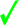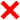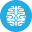# PHP Language | 10 Minute‐Test 9

Instruction

• Total number of questions : 10.
• Time alloted : 10 minutes.
• Each question carry 1 mark.
• No Negative marks
• DO NOT refresh the page.
• All the best :-).

1.

What will be the output of the following php code?

<?php

\$num  = 1;

\$num1 = 2;

print \$num + \$num1;

?>

A.
 3B.
 1+2C.
 ErrorD.
 12Correct Answer: Option A

Explanation:

The numbers inside the double quotes are considered as integers and not string, therefore the value 3 is printed and not 1+2.

2.

What will be the output of the following code?

<?php

function track() {

static \$count = 0;

\$count++;

echo \$count;

}

track();

track();

track();

?>

A.
 123B.
 111C.
 000D.
 011Correct Answer: Option A

Explanation:

Because \$count is static, it retains its previous value each time the function is executed.

3.

What is the value of \$a and \$b after the function call?

<?php

function doSomething( &\$arg ) {

\$return = \$arg;

\$arg += 1;

return \$return;

}

\$a = 3;

\$b = doSomething( \$a );

?>

A.
 a is 3 and b is 4.B.
 a is 4 and b is 3.C.
 Both are 3.D.
 Both are 4.Correct Answer: Option B

Explanation:

\$a is 4 and \$b is 3. The former because \$arg is passed by reference, the latter because the return value of the function is a copy of the initial value of the argument.

4.

A function in PHP which starts with __ (double underscore) is know as.

A.
 Magic FunctionB.
 Inbuilt FunctionC.
 Default FunctionD.
 User Defined FunctionCorrect Answer: Option A

Explanation:

PHP functions that start with a double underscore – a “__” – are called magic functions in PHP. They are functions that are always defined inside classes, and are not stand-alone functions.

5.

What will be the output of the following PHP code?

<?php

echo ucwords("i love my country");

?>

A.
 I love my countryB.
 i love my CountryC.
 I love my CountryD.
 I Love My CountryCorrect Answer: Option D

Explanation:

The ucwords() function converts the first character of each word in a string to uppercase.

6.

What will be the output of the following PHP code?

<?php

\$fruits = array ("apple", "orange", array ("pear", "mango"),

"banana");

echo (count(\$fruits, 1));

?>

A.
 3B.
 4C.
 5D.
 6Correct Answer: Option D

Explanation:

The array entity holding pear and mango is counted as an item, just as its contents are.

7.

What will be the output of the following PHP code ?

<?php

\$number = range(0, 5);

print_r (\$number);

?>

A.
 Array (  => 0  => 1  => 2  => 3  => 4  => 5 )B.
 Array (  => 0  => 0  => 0  => 0  => 0  => 0 )C.
 Array (  => 5  => 5  => 5  => 5  => 5  => 5 )D.
 Array (  => 0  => 5 )Correct Answer: Option A

Explanation:

the range() function creates an array containing a range of elements.

8.

What will be the output of the following PHP code?

<?php

\$a = array("a"=>"red", "b"=>"green", "c"=>"blue");

echo array_shift(\$a);

print_r (\$a);

?>

A.
 greenB.
 redC.
 blueD.
 none of the aboveCorrect Answer: Option B

Explanation:

The array_shift() function removes the first element from an array, and returns the value of the removed element.

9.

What will be the output of the following PHP code?

<?php

\$number = array ("4", "hello", 2);

echo (array_sum (\$number));

?>

A.
 4hello2B.
 4C.
 2D.
 6Correct Answer: Option D

Explanation:

The array_sum() function add all the values of the input array together, returning the final sum. If a string datatype is found, it’ll be ignored.

10.

Which of the following is/are the right way to declare a method?
i) function functionName() { function body }
ii) scope function functionName() { function body }
iii) method methodName() { method body }
iv) scope method methodName() { method body }

A.
 Only ii)B.
 Only iv)C.
 i) and ii)D.
 iii) and iv)Correct Answer: Option C

Explanation:

In case of public methods, you can forgo explicitly declaring the scope and just declare the method like you would a function.

Try More: Boost your Knowledge
#####Test 27

Submit your test now to view the Results and Statistics with answer explanation.